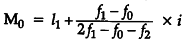# How is the Mode determined in a continuous series?

In a continuous series, the mode is determined by two methods, as given below :
(i) Inspection Method: If the items are connected in one class, we determine the modal class and then to determine the exact value, we apply the formula(ii)Grouping Method : If values are connected at more than one value, then a grouping table and an analysis table is prepared in the same way as in discrete series and to know the exact value of the mode, we apply the formula given above.